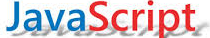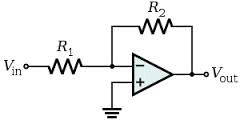## EE Calculator CollectionHere's a growing collection - just like the SPICE circuits - of JavaScript based electrical engineering calculators that you can run as is. Or, download and run them on your PC. Use them as templates for your own custom calculators.

 RC Filter, Calc fc - 1 Button Simple example that calculates fc given R and C using a single button and function. Great starter file. RC Filter, Calc R,C,fc - 3 Button Calculates fc, R or C using 3 buttons that calls three separate JavaScript functions. R Divider Calc Calculates R1, R2 or K 3 buttons that calls three separate JavaScript functions. Op Amp Gain Calculates R1, R2 or K for inverting and non-inverting amplifiers using 2 radio buttons and 3 input buttons. An alert window pops up if specified gain has incorrect polarity. Op Amp Bandwidth Calculates R2 and BW for inverting and non-inverting amplifiers. Op Amp Differential Amp Enter Gain resistors, input sign and common mode signal. Calculates signal gain and common mode gain. See how resistor mismatch causes common mode errors. Get Units of Precision Enter any units of ratio, percent, ppm, dB or Nbits. Then calculate its equivalent in all other units. A single function handles multiple buttons by passing a text parameter to the routine. Step Response of RC Filter Creates a textbox on the form and displays the step response of an RC filter. A set of time and voltage pairs is calculated and writen to the textbox. This textbox will be useful later when creating custom SPICE models. Create Capacitor SPICE Model Enter your capacitor parameters. The code creates a SPICE model and snds it to a TextBoxa ready for you to copy into a SPICE subcircuit. Op Amp Builder - Level 1 The code creates a simple Op Amp SPICE Macro-Model that models Gain, Bandwidth and Input/Output Resistance. (See Cap model for JavaScript form details). Op Amp Builder - Level 2 Create create an intermediate Op Amp SPICE Macro-Model that models Slew Rate Limiting, Output voltage/current limits and overshoot/ringing. (See Cap model for JavaScript form details). Draw Rectangle on Canvas Drawing on the canvas was far simpler than I thought. See how to take user inputs defining a rectange and create a line drawing on the canvas element.. Draw Sine Wave on Canvas Take user inputs defining a sine wave and transform it into x and y pixel coordinates..